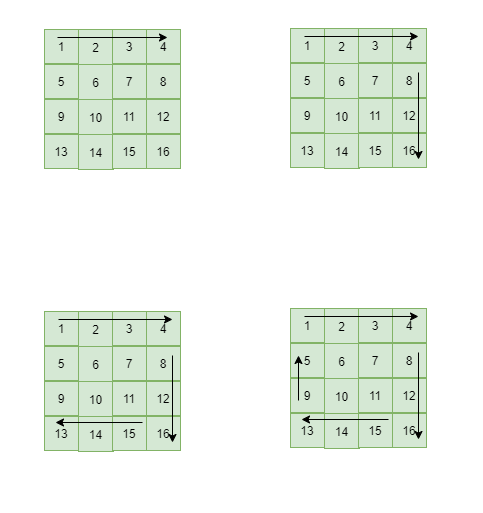• 累计撰写 145 篇文章
• 累计创建 37 个标签
• 累计收到 18 条评论

### 目 录CONTENT# 剑指Offer【19】-- 顺时针打印矩阵2020-11-28 / 0 评论 / 0 点赞 / 255 阅读 / 0 字

[toc]

# 思路与解析

• 从第一个行第一个开始打印，向左边界遍历到右边界，之后将上边界加上1（因为已经遍历完成上边界一行），判断上边界加上一之后是否大于下边界，如果是则调出。
• 之后执行类型操作，从上到下，从右到左，从下到上。import java.util.ArrayList;
public class Solution {
public ArrayList<Integer> printMatrix(int[][] matrix) {
ArrayList<Integer> results = new ArrayList();
if (matrix != null && matrix.length > 0) {
int left = 0;
int right = matrix.length - 1;
int up = 0;
int down = matrix.length - 1;
int i;
while (true) {
for (i = left; i <= right; i++) {
}
if ((++up) > down) {
break;
}
for (i = up; i <= down; i++) {
}
if (--right < left) {
break;
}
for(i=right;i>=left;i--){
}
if(--down<up){
break;
}
for(i=down;i>=up;i--){
}
if(++left>right){
break;
}
}
}
return results;
}
}0